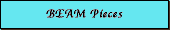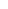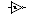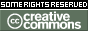HomeSourcesPart descriptionsElectrical partsPassive componentsResistorsResistor part codesCapacitorsCapacitor part codesThrough-holeSurface mountBatteriesBatteries comparedSemiconductorsIntegrated circuitsPackagesMechanical partsMisc. partsAssembly descriptions

BEAM Pieces is a BEAM Reference Library site.

Capacitor part codes
Breaking the code, part 2

Large capacitors have the value printed plainly on them, such as 10.uF (Ten Micro Farads) but smaller disk types along with plastic film types often have just two or three numbers on them.

First, most will have three numbers, but sometimes there are just two numbers. These are read as Pico-Farads. An example: 47 printed on a small disk can be assumed to be 47 Pico-Farads (or 47 puff as some like to say)

Now, what about the three numbers? It is somewhat similar to the resistor code. The first two are the 1st and 2nd significant digits and the third is a multiplier code. Most of the time the last digit tells you how many zeros to write after the first two digits, but the standard (EIA standard RS-198) has a couple of curves that you probably will never see. But just to be complete here it is in a table.

Table 1 Digit multipliers

Third digit

Multiplier (this times the first two digits
gives you the value in Pico-Farads)

0

1

1

10

2

100

3

1,000

4

10,000

5

100,000

6 not used

7 not used

8

.01

9

.1

Now for an example: A capacitor marked 104 is 10 with 4 more zeros or 100,000pF which is otherwise referred to as a .1 uF capacitor.

Just to confuse you some more there is sometimes a tolerance code given by a single letter. I don't know why they were picked in the order they are, except that it kind of follows the middle row of keys on a typewriter.

So a 103J is a 10,000 pF with +/-5% tolerance

Table 2 Letter tolerance code

Letter symbol

Tolerance of capacitor

B

+/- 0.10%

C

+/- 0.25%

D

+/- 0.5%

E

+/- 0.5%

F

+/- 1%

G

+/- 2%

H

+/- 3%

J

+/- 5%

K

+/- 10%

M

+/- 20%

N

+/- 0.05%

P

+100% ,-0%

Z

+80%, -20%

Now to really complicate things there is sometimes a letter-number-letter (like Z5U) code that gives information. Table 3 shows how to read these cryptic codes. A 224 Z5U would be a 220,000 pF (or .22 uF) cap with a low temperature rating of -10 deg C a high temperature rating of +85 Deg C and a tolerance of +22%,-56%.

Table 3 Dielectric codes

First symbol
(a letter)

Low temperature requirement

Second symbol
(a number)

High Temperature requirement

Third Symbol
(a letter)

MAX. Capacitance change over temperature

Z

+10 deg. C

2

+45 deg. C

A

+1.0%

Y

-30 deg. C

4

+65 deg. C

B

+/- 1.5%

X

-55 deg. C

5

+85 deg. C

C

+/- 2.2%

6

+105 deg. C

D

+/- 3.3%

7

+125 deg. C

E

+/- 4.7%

F

+/- 7.5%

P

+/- 10.0%

R

+/- 15.0%

S

+/- 22.0%

T

+22%, -33%

U

+22%, -56%

V

+22%, -82%

There are some Capacitor color codes - the last dot is the tolerance code where brown is +/-1% red +/-2% as in the resistor color code with two exceptions black is +/- 20% and white is +/- 10% going backward the three dots to the left of the tolerance dot form the value in pF There will be two or three more color dots before the value but they mean different things about temperature range and coefficient depend which one of three systems is used - so I will leave it out for now unless some one asks.

There are two more number systems seen on caps. The first one can be recognized as the EIA because it starts with an R.

R DM 15 F 471(R) J 5 O (C)

The above number means the following

 R tells us this is an EIA code is the case code - DM is a dipped case style, CM would be a molded case style is the case size code - if anyone asks I will put up a table for this is the characteristic code from table 4 the R is a decimal point when used (not often), the first two digits form the significant value and the third is the multiplier; this is a 470pF part is the capacitance tolerance code as given in table 2 above; J is a 5% part is the DC working voltage in hundreds of volts (EIA only) thus 500V is the temperature range from table 5 tells us the leads are crimped where a S would tell us they are straight.

This next one is the Military code

CM 15 B D 332 K N 3

 CM is the case code - DM is a dipped case style, CM would be a molded case style is the case size code - if anyone asks I will put up a table for this characteristic code tells us it doesn't have a drift specified (from table 4) is the Military voltage code from table 6 tells us that it is 3,300pF tells us from table 2 that this is a 10% part gives us our temperature range of -55 to 85 �C from table 5 Vibration grade 3 tells us 20g at 10 to 2,000 hz for 12 hours (1 is 10G at 10 to 55 Hz for 4.5 hours)

Table 4 characteristic codes

EIA or MIL characteristic code

Maximum capacitance drift

Maximum range of Temp coefficient

B

Not specified

Not specified

C

+/-(0.5% + 0.1pF)

+/- 200 ppm/�C

D

+/-(0.3% + 0.1pF)

+/- 100 ppm/�C

E

+/-(0.1% + 0.1pF)

-20 to +100 ppm/�C

F

+/-(0.05% + 0.1pF)

0 to +70 ppm/�C

Table 5 Temperature range

M

-55 to 70 �C

N

-55 to 85 �C

O

-55 to 125 �C

P

-55 to 150 �C

Table 6 Mil voltage range code in volts

A

100

B

250

C

300

D

500

E

600

F

1,000

G

1,200

H

1,500

J

2,000

K

2,500

L

3,000

M

4,000

N

5,000

P

6,000

Q

8,000

R

10,000

S

12,000

T

15,000

U

20,000

V

25,000

W

30,000

X

35,000

Surface-mount capacitors
Based on a list posting by Vincent Chin...

Most manufacturers mark their components but often leave capacitors (with the exception of tantalum capacitors) unmarked. A basic three symbol code is used on some capacitors. The first two marked digits are the first two digits of the value, and the third digit is a multiplier. This marking system is exactly the same as the marking system used for ceramic capacitors. All the values marked are in picoFarads.

 Marked Value 123 12000 pF / 12 nF 122 1200 pF / 1.2 nF 121 120 pF / 0.12 nF 654 650000 pF / 650 nF / 0.65 uF

A two symbol system is also used (this is more common for capacitors). The first symbol in the two digit system is a letter representing a number value; the second symbol is the multiplier (power of ten). All the values marked are in picoFarads.

A = 1.0
B = 1.1
C = 1.2
D = 1.3
E = 1.5
F = 1.6
G = 1.8
H = 2
J = 2.2
K = 2.4
L = 2.7
M = 3
N = 3.3
P = 3.6
Q = 3.9
R = 4.3
S = 4.7
T = 5.1
U = 5.6
V = 6.2
W = 6.8
X = 7.5
Y = 8.2
Z = 9.1

a = 2.5
b = 3.5
d = 4
e = 4.5
f = 5
m = 6
n = 7
t = 8
y = 9

0 = x1
1 = x10
2 = x100
3 = x1000
4 = x10000, etc.

Aluminium electrolytic SMD capacitors may have a three symbol code with numbers to indicate capacitance in microFarads (uF) and a letter to indicate the voltage rating. The position of the letter indicates the decimal point position in the capacitance value. The letter code is as shown here:

C = 6.3 V
D = 10 V
E = 16 V
F = 25 V
G = 40 V
H = 63 V

Thus "F47"=0.47uF / 25v. Note that the C type is best for BEAM, but they are hard to come by; SMD caps are most commonly found in E or F ratings.

 For more information... There's an on-line capacitor value calculator here. Another, more-graphical one used to be here (site offline 4/18/03).

 SitemapSearchLegalitiesPage authors: Eric Seale & Vincent Chin This page was last updated onThis work is licensed under a Creative Commons License.